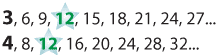Addition of fractions with different and unrelated denominators

To add fractions with different denominators we first convert to equivalent fractions with the same denominator.

\begin{align}\frac{2}{3} + \frac{9}{12} &= \frac{8}{12} + \frac{9}{12} \text{(convert to equivalent fractions with the same denominator)} \\&= \frac{17}{12} \\&= 1\frac{5}{12} \text{(convert the improper fraction to a mixed number)}\end{align}

Note that both 3 and 4 are factors of 12 and 12 is the Lowest Common Multiple (LCM) of 3 and 4.Detailed description